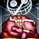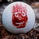# A Fibonacci Moving Averages Ribbon Using One Plot

1760 뷰
The following script aims to visually reproduce a Fibonacci moving averages ribbon by only using one plot function, the period of the moving average is determined by a number of the Fibonacci sequence. This trick is made possible by computing the value of a moving average at time t with a period determined by a periodic Fibonacci sequence and using plot.style_circles as plot style.

Settings

• From Fibonacci Number: Determine the nth Fibonacci number to be used as the lowest moving average period
• To Fibonacci Number: Determine the nth Fibonacci number to be used as the highest moving average period, capped at 19 to avoid errors. If you still have errors lower the value.
• Src : input series of the moving average.

Details

The first thing we must do is to compute a periodic (repeating) linear sequence n from the number in From Fibonacci Number to the number in To Fibonacci Number, then we get the nth Fibonacci number from the previous sequence using Binet's formula.

To get the moving average we first compute the fib period momentum of the cumulative sum of Src, where fib is the current number of our periodic Fibonnacci sequence, we then divide the result by fib.

If we zoom in we can indeed see that there is only one point per bar.

However, zooming out and using a different color for each point allows us to get something visually similar to a ribbon. Adding more plots would create a visually more accurate result.

This trick is not the most useful in the world, but let's imagine you want to plot a massive ribbon consisting of 1000 moving averages with periods that can be determined by a specific sequence, using this trick would allow you to have the effect of 1000 plots while keeping your script relatively efficient, altho ribbons consisting of lots of moving averages are rarely easy to visualize.
Patreon: https://www.patreon.com/alexgrover

You can also check out some of the indicators I made for luxalgo : https://www.tradingview.com/u/LuxAlgo/#published-scripts

## 코멘트Reducing the number of plots is a very useful trick in this world atm.lol
응답Sweet ! Do fib circles next, I'm too stupid still... :)
응답alexgrover
@alexgrover, I just need the formula for drawing the other side of the ellipse and offset the plot forward
I have some auto gann stuff that I have done so I'm not entirely stupid... and i'm sure i'll work it out with enough head banging using your ellipses script as a basis... Could I perhaps DM you ?
응답
프로화일 프로화일설정 계정 및 빌링 리퍼드 프렌즈 코인 나의 서포트 티켓 헬프 센터 공개아이디어 팔로어 팔로잉 비밀메시지 채팅 로그아웃